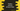# Convert string to float in python# Convert string to float in python :

Sometimes, we need to convert a string to a float value. For example, if you are receiving float data in string format from the server and if you want to do any arithmetic operations on them, you need to convert them to float first.

For example, let’s take a look at the below program :

As you can see, we are getting one ‘TypeError’ because the first variable ‘a’ is a string and we are trying to add it with ‘b’, which is a float. We can’t add one string with a float. To handle errors like the above one, we need to convert the string value to a float value before performing any arithmetic operation.

Note that this method will work only if any floating value is represented as a string. If the value is not a float and it is actually a different string like “null”, it will fail.

So, we need to convert the string to a float before using it with any arithmetic operations in the above example. In this post, I will show you how to do this conversion.

## Simple program to convert a string to float :

Let’s try to change the above example and convert the string to a float before doing the addition. String to float conversion in python is really easy. We don’t need to import any extra module and we don’t need any complex procedure to do it. Just wrap the string value with ‘float()’ method and the conversion will be done.

This method is defined as float(str). ’str’ is the string parameter need to pass to this method.

Here, we have converted the string ‘a’ to float using the ‘float()’ method and add it with ‘b’. As you can see that no error was raised this time.

## Converting all items in a list :

This is another example of a string to float conversion. Suppose we have one list with float numbers stored as a string. If our problem is to find out the sum of all of these numbers, we need to convert them to float before doing the addition operation. Or, for any other arithmetic operation, we need to convert them to float first.

To find out the total sum of all values in the list, we can use one for loop to iterate over the list and convert each string value to float one by one and add it to a final variable. We are using the same ‘float()’ method to do the string-float conversion.

We can use one for loop to iterate over the list. You can find the below examples here on Github.

``````num_list = ["1.11","2.22","3.33"]

sum = 0

for x in num_list :
sum += float(x)

print(sum)``````

It will print 6.66 as output. We can also convert these elements and create one list of floating values like below :

``````num_list = ["1.11","2.22","3.33"]

float_list = [ float(x) for x in num_list]

print(num_list)
print(float_list)``````

As you can see that the original list with string values are converted to a list with floating values.

## Conclusion :

In this tutorial, we have learned how to convert a string value to float. If we are not sure about the receiving values, it is a good coding practice to convert it before using. Try to run the examples and drop one comment below if you have any queries.